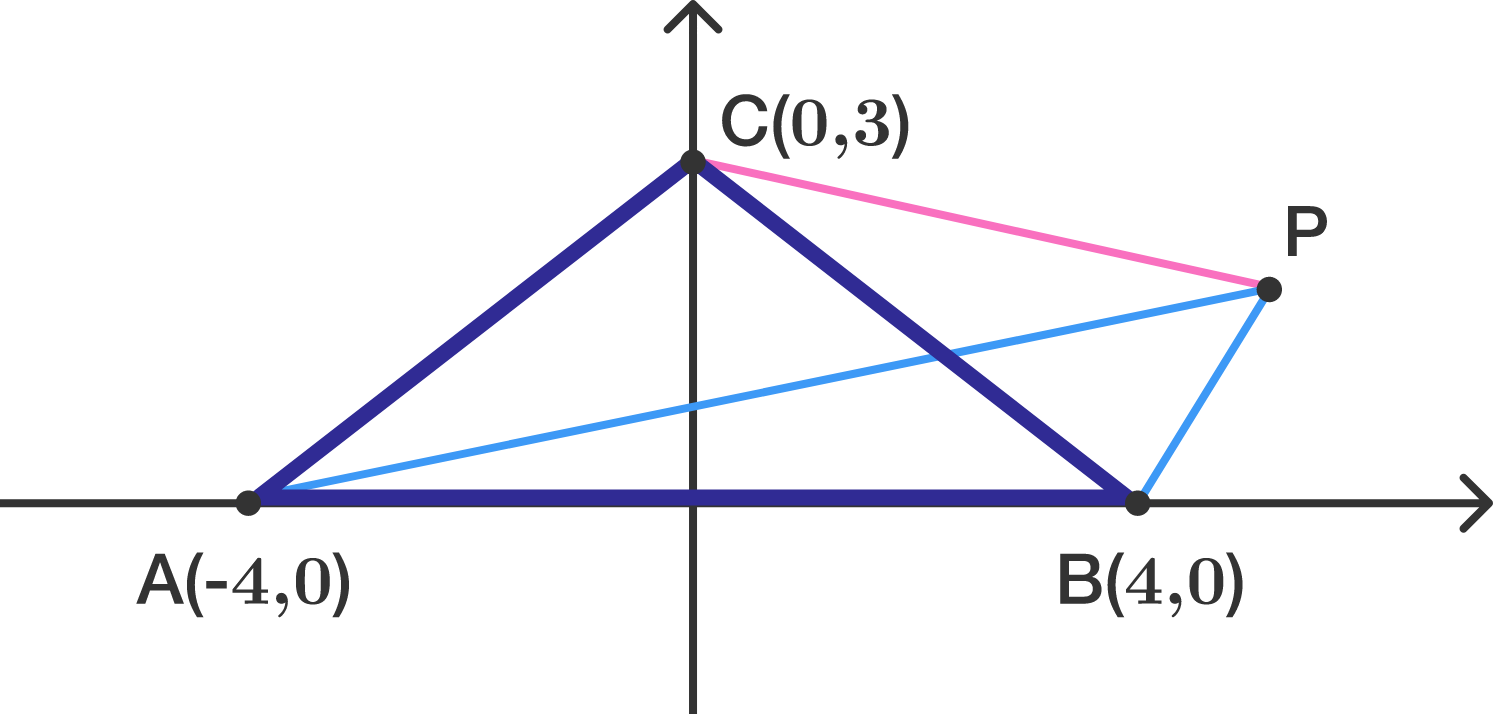# Bending A Triangle

Geometry Level 2Triangle $ABC$ has coodinates $A= (-4, 0)$, $B= (4 , 0)$, and $C= (0 , 3)$.

Let $P$ be the point in the first quadrant such that $\triangle ABP$ has half the area of $\triangle ABC$ but both triangles have the same perimeter.

What is the length of $CP?$ If your solution is in a form of $\sqrt{d}$, submit $d$ as the answer.

×